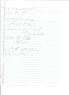# Finding The Solution of A Differential Equation

Bashyboy

## Homework Statement

y' + 3y = t + exp(2t)

## The Attempt at a Solution

My solution is y = t/3 + exp(-2t) -1/9 + c. The solution in the back of the book is y = cexp(-3t) + (t/3) - (1/9) + exp(-2t). How come the two solutions differ so vastly? I followed the procedure outlined in the textbook precisely.

Last edited:

Homework Helper

## Homework Statement

y' + 3y = t + exp(2t)

## The Attempt at a Solution

My solution is y = t/3 + exp(-2t) -1/9 + c. The solution in the back of the book is y = cexp(-3t) + (t/3) - (1/9) + exp(-2t).

The solution I'm getting is :

##y(t) = \frac{c}{e^{3 t}} + \frac{t}{3} + \frac{e^{2 t}}{5} - \frac{1}{9}##

You should check your work over again I think. You may have made a slight error.

Last edited:
Bashyboy
I got the exact same answer. I attached my work as a file. According to my textbook, the solution to this sort of differential equation is:

$y = \frac{1}{\mu (t)} \int \mu (t) g(t) dt + c$, where $\mu (t) = e^{\int p(t)dt}$

#### Attachments

•DE.jpg
14 KB · Views: 368
Homework Helper
I got the exact same answer. I attached my work as a file. According to my textbook, the solution to this sort of differential equation is:

$y = \frac{1}{\mu (t)} \int \mu (t) g(t) dt + c$, where $\mu (t) = e^{\int p(t)dt}$

Yes your book is 100% correct ( Barring I'd leave constants all the way until the end ), but don't neglect the ##e^{3t}## in the denominator. It will vary the value of ##c## if you're ever given an initial value problem.

Last edited:
Bashyboy
Oh, I see. So exp(-3t) would multiply the c, after I did the integration.

Homework Helper
Oh, I see. So exp(-3t) would multiply the c, after I did the integration.

It will divide it actually. After evaluating the integrals I got this :

##e^{3t}y(t) = e^{3t} ( \frac{e^{2t}}{5} + \frac{t}{3} - \frac{1}{9} ) + c##